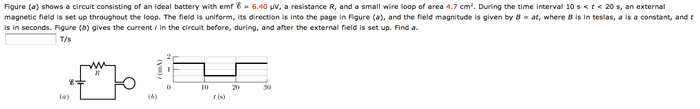# Induced current in a loop circuit magnetic field

## Homework Statement## Homework Equations

I know this is supposed to use Lenz's law, but I don't really understand it

V=IR

## The Attempt at a Solution

I calculated the resistance of the circuit to be 6.4e-6 / .0015 = 0.00426666667, but that's as far as i know what to do